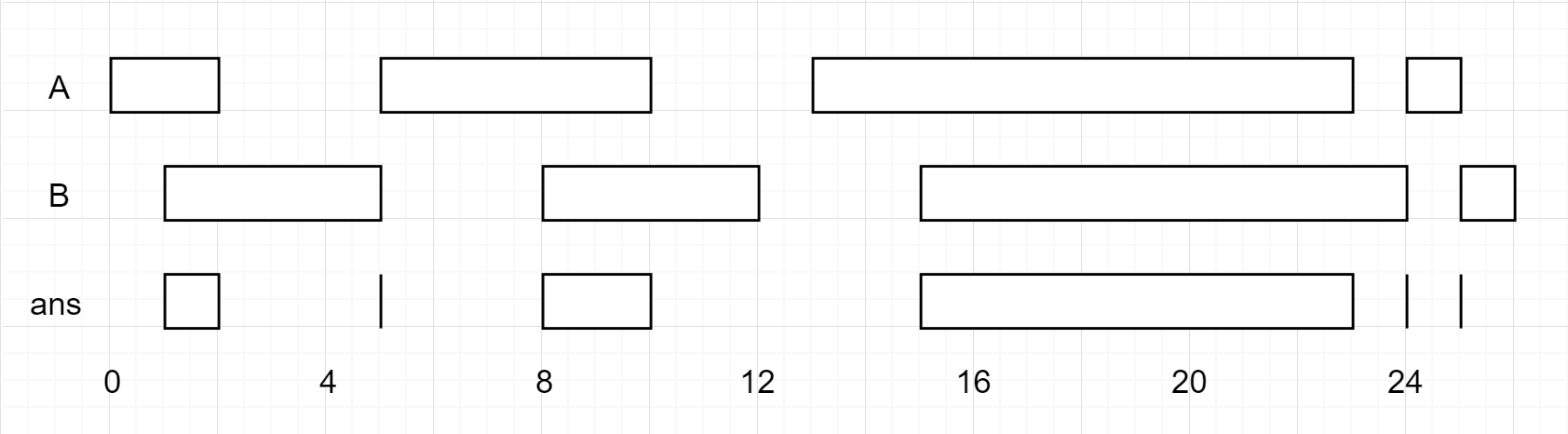## C# || How To Find Interval List Intersections From Two Arrays Using C#The following is a module with functions which demonstrates how to find interval list intersections from two arrays using C#.

1. Interval Intersection – Problem Statement

You are given two lists of closed intervals, firstList and secondList, where firstList[i] = [starti, endi] and secondList[j] = [startj, endj]. Each list of intervals is pairwise disjoint and in sorted order.

Return the intersection of these two interval lists.

A closed interval [a, b] (with a <= b) denotes the set of real numbers x with a <= x <= b.

The intersection of two closed intervals is a set of real numbers that are either empty or represented as a closed interval. For example, the intersection of [1, 3] and [2, 4] is [2, 3].

Example 1:``` Input: firstList = [[0,2],[5,10],[13,23],[24,25]], secondList = [[1,5],[8,12],[15,24],[25,26]] Output: [[1,2],[5,5],[8,10],[15,23],[24,24],[25,25]] ```

Example 2:

``` Input: firstList = [[1,3],[5,9]], secondList = [] Output: [] ```

Example 3:

``` Input: firstList = [], secondList = [[4,8],[10,12]] Output: [] ```

Example 4:

``` Input: firstList = [[1,7]], secondList = [[3,10]] Output: [[3,7]] ```

2. Interval Intersection – Solution

The following is a solution which demonstrates how to find interval list intersections from two arrays.

This solution uses the two pointer technique when looking for valid interval intersections.

``` 2. Interval Intersection - Solution C# // ============================================================================ // Author: Kenneth Perkins // Date: Nov 23, 2021 // Taken From: http://programmingnotes.org/ // File: Solution.cs // Description: Demonstrates how to find interval list intersections // ============================================================================ public class Solution { public int[][] IntervalIntersection(int[][] firstList, int[][] secondList) { var result = new List<int[]>(); // Go through first and second array looking for range intersection var indexFirst = 0; var indexSecond = 0; while (indexFirst < firstList.Length && indexSecond < secondList.Length) { // Get the first and second array var first = firstList[indexFirst]; var second = secondList[indexSecond]; // Check to see if this is a valid intersection if (first >= second && first <= second) { // Get starting and ending range from the two arrays var start = Math.Max(first, second); var end = Math.Min(first, second); result.Add(new int[] {start, end}); } // Increment array index depending on which range is completely satisfied if (first < second) { ++indexFirst; } else { ++indexSecond; } } return result.ToArray(); } }// http://programmingnotes.org/ 123456789101112131415161718192021222324252627282930313233343536 // ============================================================================//    Author: Kenneth Perkins//    Date:   Nov 23, 2021//    Taken From: http://programmingnotes.org///    File:  Solution.cs//    Description: Demonstrates how to find interval list intersections// ============================================================================public class Solution {    public int[][] IntervalIntersection(int[][] firstList, int[][] secondList) {        var result = new List<int[]>();         // Go through first and second array looking for range intersection        var indexFirst = 0;        var indexSecond = 0;        while (indexFirst < firstList.Length && indexSecond < secondList.Length) {            // Get the first and second array            var first = firstList[indexFirst];            var second = secondList[indexSecond];             // Check to see if this is a valid intersection            if (first >= second && first <= second) {                // Get starting and ending range from the two arrays                var start = Math.Max(first, second);                var end = Math.Min(first, second);                result.Add(new int[] {start, end});            }            // Increment array index depending on which range is completely satisfied            if (first < second) {                ++indexFirst;            } else {                ++indexSecond;            }        }        return result.ToArray();    }}// http://programmingnotes.org/ ```

QUICK NOTES:
The highlighted lines are sections of interest to look out for.

The code is heavily commented, so no further insight is necessary. If you have any questions, feel free to leave a comment below.

Once compiled, you should get this as your output for the example cases:

``` [[1,2],[5,5],[8,10],[15,23],[24,24],[25,25]] [] [] [[3,7]] ```